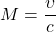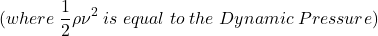# Stagnation Pressure – Uses and Real Life Applications

#### Engineering

Stagnation pressure is the fluid pressure that is measured while an initially flowing fluid is fully at rest. The fluid still holds mechanical energy through pressure despite having no movement. In this article, you will learn how to determine stagnation pressure, its use in incompressible and compressible flow, and applications where stagnation pressure is important.

## Determining Stagnation Pressure

Stagnation pressure is a value that can be measured or calculated. Moreover, one of the most common ways to measure it is using a device called a Pitot tube. This device has multiple entry points, there is a main entrance on the front with no exit point that measures the stagnation pressure. However, there are also side holes that measure static pressure. This can then be used to determine the dynamic pressure of the system by taking the difference in them.

A stagnation point is a point where the liquid is at rest. the point where stagnation pressure is measured at this point. Incidentally this is the point in which the fluid only holds pressure energy. Even objects used to be as flow efficient as possible still hold stagnation points. Likewise rounded surfaces will have some stagnation point on the surface at any time a fluid is flowing over it. Stagnation points cause inefficiencies in flow, so when trying for maximum efficiency its best to minimize the amount of possible stagnation points.

## Determining Whether the Flow is Incompressible or Compressible

It is also important when calculating the stagnation pressure to determine if the flow is incompressible or compressible. In order to determine which kind of flow it is, the Mach number(M) can be applied. When the calculated Mach number is less than 0.3 then the material is incompressible. Likewise if it’s greater than 0.3 it is compressible. Flow velocity(υ) is divided by the speed of sound in the material(c).If the fluid is determined to be incompressible the stagnation point also needs to be known. Using the point to know the relative velocity of the fluid will help give you your dynamic flow. Likewise with compressible flow the Temperatures’ and ratio of specific heats need to be know to fully calculate the value.

## Pressure in Incompressible Flow

When dealing with an incompressible fluid, there is a fairly simple way to calculate it rooting from the Bernoulli equation. This method uses the initial and final pressure(P), density(ρ), velocity of the fluid(ν), gravitational pull(g) and the height(h)Because in this circumstance there is no change in height between the initial and the final, users can drop the ρgh portion of the equation, thus simplifying it into a more usable equation.Because stagnation pressure happens when the fluid is at rest, the right side of the equation turns into just stagnation pressure because the velocity is 0. The left side holds the initial static pressure and the dynamic pressure. Density(ρ) and the speed of the fluid(ν) are relative to the stagnation point.Incompressible flow does not necessarily mean incompressible fluid. However the flow of the fluid is incompressible, giving it a constant density throughout the measured flow.

## Stagnation Pressure in Compressible Flow

Compressible flow is not able to measure the dynamic pressure in the same way because the density changes throughout the flow. Taking into account the stagnation pressure, static pressure, the ratio of specific heats(γ), and the previously calculated Mach number(M) produces the following equation:If the application happens in an isentropic setting, the stagnation pressure results from the ratio of temperatures.